# Geometry

#### Coordinate Geometry - 2

Special Triangles

Special Triangles

1. Equilateral Triangle: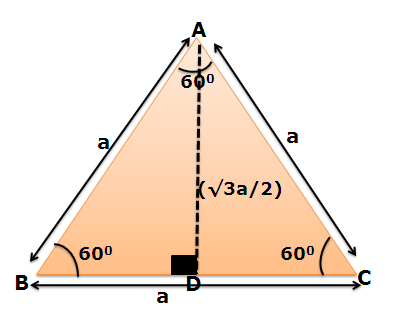In an equilateral triangle, centroid, orthocenter , in centre and circum centre are all coincident i.e. the medians, altitudes, angle bisectors and perpendicular bisectors are all the same.

So, AD will be the median as well as an altitude as well as an angle bisector and also the perpendicular bisector.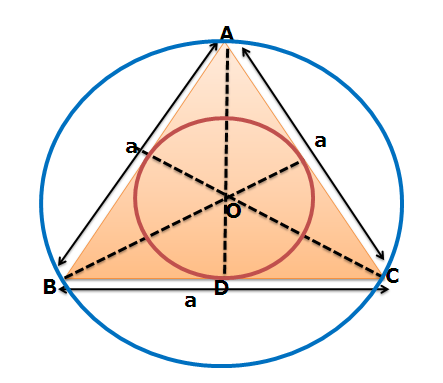As we know that centroid of a triangle divides the medians in the ratio of 2:1. So, AO : OD is 2:1

Thus, AO = (2/3)*(√3a/2) = (a/√3)

OD = (1/3)*(√3a/2) = (a/2√3)

As we see that OD is the radius of the incircle and AO is the radius of the circumcircle circumscribing the triangle.

r = OD = (a/2√3)

R = AO = (a/√3)

Important:

1. The triangle of greatest area among all those with given perimeter is equilateral.

2. The triangle of largest area of all those inscribed in a given circle is equilateral; and the triangle of smallest area of all those circumscribed around a given circle is equilateral.

Q1. ABCD is a square and ΔAEB is an equilateral triangle. What is the measure of  DEC?

1. 600         2. 750            3. 1200              4. 1500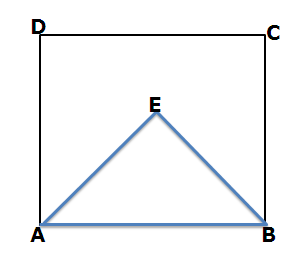Solution:

As ΔAEB is on base AB which is a side of a square. So AB = AD = CD = BC = AE = EB.

Now, ADE = AED = 750 { Angles opposite to equal sides}

EDC = 90 – 75 = 150

Similarly  ECD = 150 .  Hence  DEC = 1800 – 150 -150 = 1500 { Angle sum property}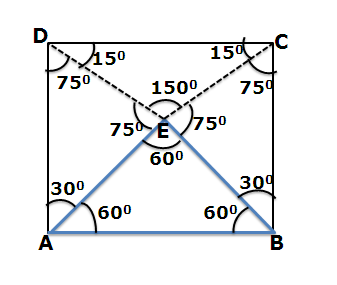Q2. The distances of a point P inside an equilateral triangle from the three sides are 5, 6 and 7 units. What is  the perimeter of the triangle?

Solution: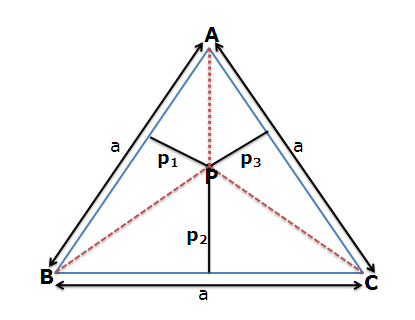Let the distances of point P from the three sides be p1,p2 and p3.

Area of ΔABC = Area(ΔABP) + Area(ΔBCP)+ Area(ΔCAP)Q3.  In the figure shown, triangle is equilateral with side length ‘a’ units. Circles are inscribed inside the triangle in the manner shown. What is the area of the region inside the triangle but outside the circles?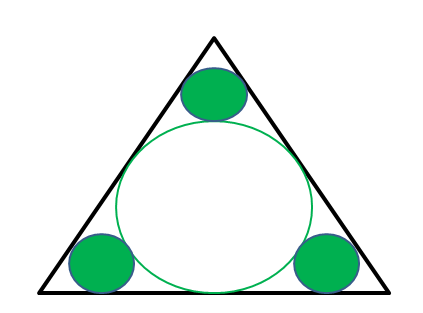Solution:

As three smaller circles are symmetrical, they have the same radius and so the same area. Ignoring the two smaller circles, we get the figure as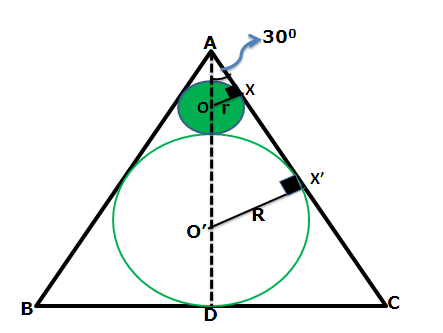In ΔAOX,   OAX = 300, so OX/OA = sin(300)  or OA = 2r (1)

Similarly in ΔAO’X’ , O’AX’ = 300, so O’X’/AO’ = sin(300) or  AO’ = 2R (2)

But AO’ = AO + OO’ = 2r + r + R = 3r + R  (3)

From (2) and (3)

3r + R = 2R     or r = R/3

In an equilateral triangle in-radius =R = (a/2√3)Q4. Three circles of radius 1 unit each touch each other as shown in the figure. What is the area of the shaded region?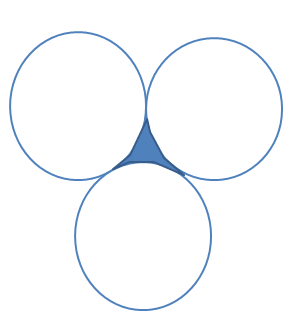Solution : If we join the centres of the circles, we get an equilateral triangle of side length 2 units.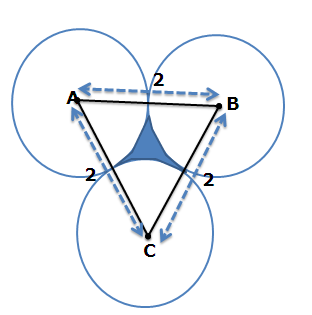Area of shaded region = Area of equilateral triangle – Area of three sectors

Now each of these sectors subtend an angle of 600 at the centre.Q5. In the given figure , ABCDEF is a regular hexagon of side length 2 units inside which two circles are inscribed. Find the area of the shaded region?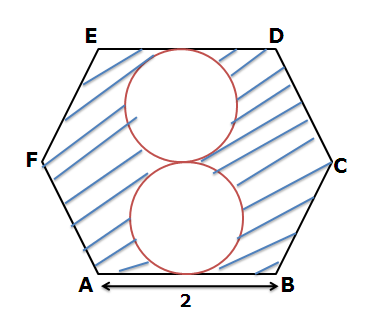Solution:  If we closely look at a regular hexagon then we find that it is made up of 6 equilateral triangles.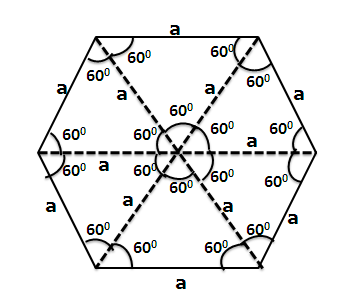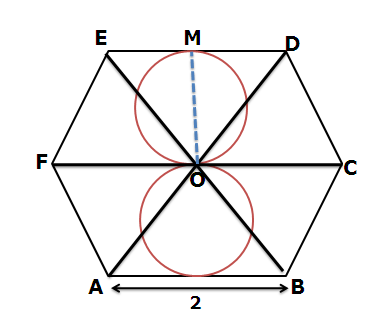Here OM is the diameter of the circle and also is the altitude of ΔEOD.Isosceles Triangle: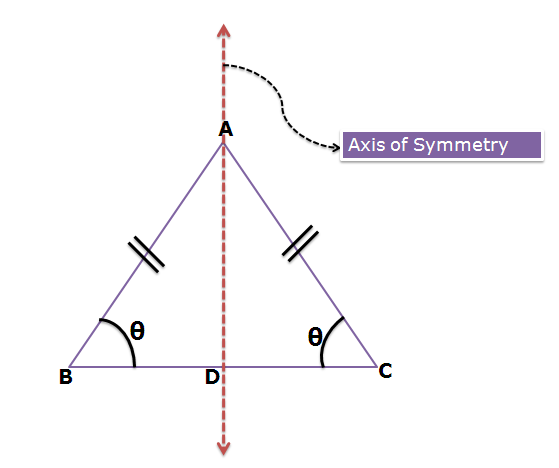In an isosceles triangle, two sides are equal and angles opposite to these equal sides are also equal.

Here AD represents the axis of symmetry, i.e. AD is the median, altitude, angle bisector and also the perpendicular bisector. This axis of symmetry goes through the vertex that is between the equal sides and also goes through the midpoint of the distinct side.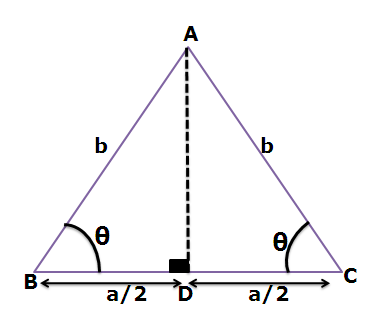AD is the altitude as well as the median, so it is perpendicular to BC and also bisects it.

Using Pythagoras theorem in ΔABD, we getSolution: An isosceles right is a triangle with two sides equal and two angles equal where as the non equal angle is equal to 900 . Let the equal sides be ‘a’ units and so the hypotenuse becomes ‘a√2’ units.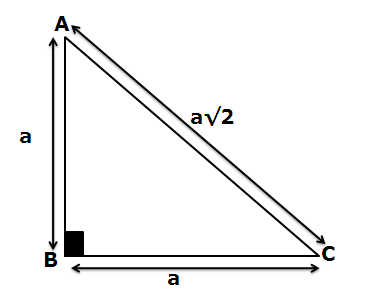Perimeter of triangle = a + a + a√2 = 2a + a√2 = 2p (given)Right Triangle: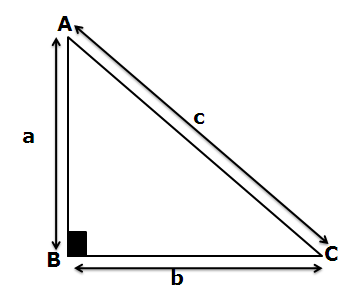A right angled triangle has one angle equal to 900. The side opposite to the right angle is known as the hypotenuse and the sides adjacent to the right angle are known as legs.

Pythagoras Theorem:

Pythagoras theorem states that  AB2 + BC2 = AC2

Three numbers a,b,c which satisfy the condition a2 + b2 = c2 are known to form Pythagorean triplets. Some of the common such triplets are (3,4,5), (5,12,13), (7,24,25) , (9,40,41), (8,15,17), (11,60,61) , (20,21,29) etc.

Area of right triangle = ½ *a*b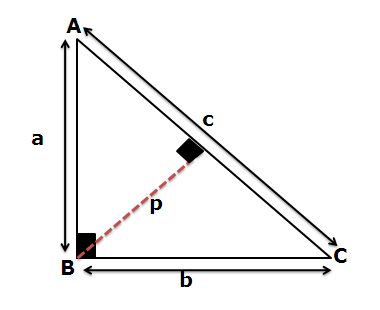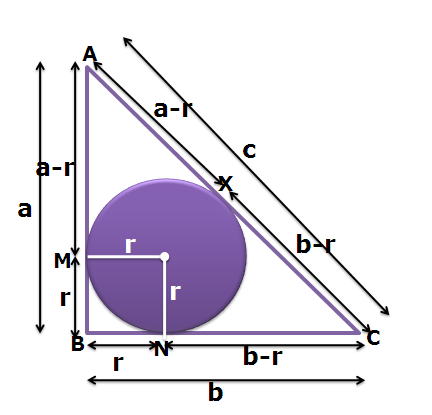As shown in the figure, if the in-radius is equal to ‘r’ units, then AM = a-r . Similarly CN = b-r

Tangents drawn from an external point to a circle are equal in length. Therefore AM = AX = a-r  and CN = CX = b-r

Also AC = AX + CX

c = a-r + b-r

Or  r= a+b-c/2

where a , b are the lengths of the legs and c is the length of the hypotenuse.

Property: - The midpoint of the hypotenuse is equidistant from the three vertices of a right angled triangle.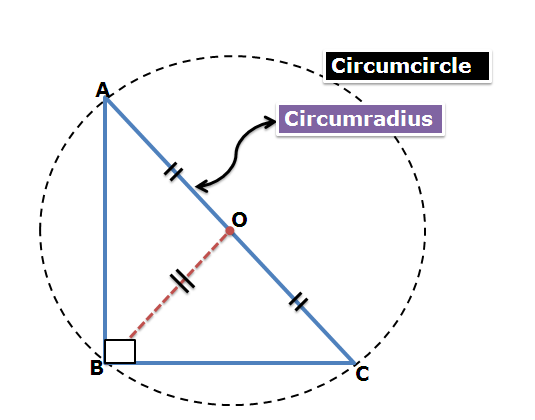Here O is the midpoint of AC. So AO = OB = OC.

Thus, with O as the centre we can draw a circle of radius R= AO = OB = OC which will pass through the three vertices A, B and C. So, such a circle which passes through the three vertices of a triangle is known as the circumcircle.

Thus, the midpoint of hypotenuse is the centre of the circumcircle and whose radius is equal to half of the hypotenuse.

Thus, R = AC/2

Q7. In the given figure all the lengths are integers and AD is perpendicular to BC. What is the ratio of in-radius of ΔABD to that of ΔADC?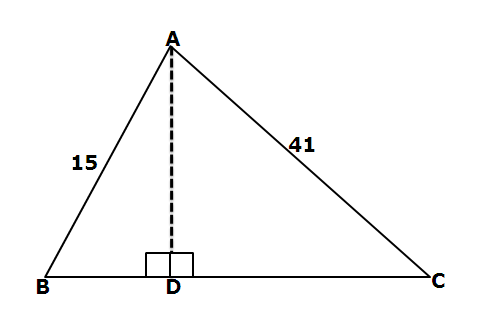So  breaking 1456 into product of two numbers such that the larger of the numbers is less than 56

1456 = 28*52 = (x-y)(x+y)

Thus,  x+y = 52     and x-y = 28

So, x = 40  and y = 12

So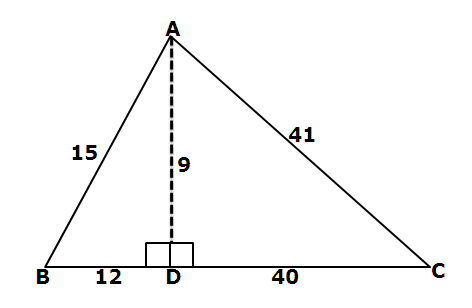Q8.   ABC is an isosceles right triangle ,right angled at A. A semicircle with centre at O is drawn inside the triangle such that AB and AC are tangent to it and the diameter of the semicircle lies along BC. What is the area of the semicircle?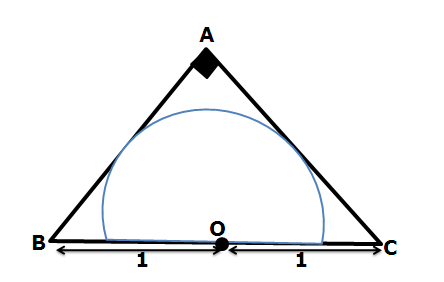Solution: As ΔABC is an isosceles right triangle, with BC = 2 units. So AB = AC = √2 units. Now as OM is perpendicular to AB and ON is perpendicular to AC, therefore OMAN is a square with side length of ‘r’ units.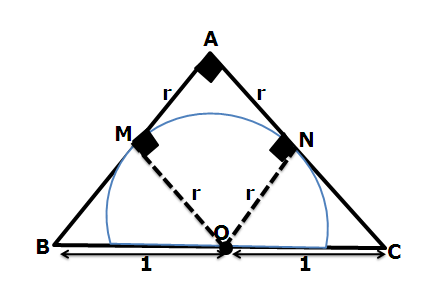AC = √2 units, AN = r units  So CN = √2 – r

In ΔONC

ON = r , OC = 1  and CN = √2 – r

Using Pythagoras theorem, we getQ9. ΔABC is right angled at B. AB= 5 units and BC = 12 units. If AD is the angle bisector of  BAC, then the length of AD is -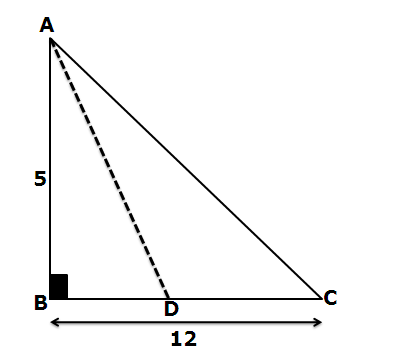Solution: AB= 5 units, BC = 12  units. So, AC = 13 units { By Pythagoras theorem}

Now AD is the angle bisector of  BAC

Angle Bisector Property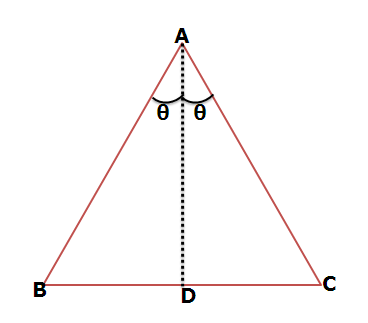So applying the above property we get

BD: DC = 5: 13Q10. A square is inscribed inside a right triangle as shown in the figure. If AB = 15 and BC = 8units. What is the side length of the square?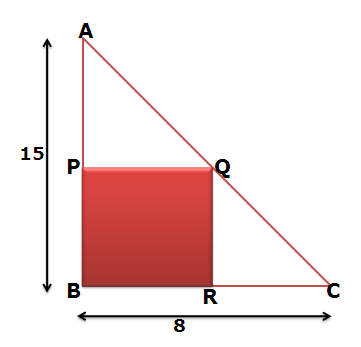Solution: Let the side length of square be ‘x’ units.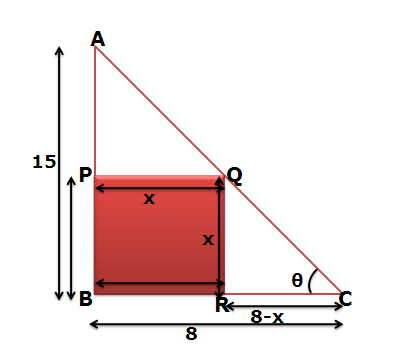In ΔABC, tanθ = AB/BC = 15/8          (1)

In ΔQRC, tanθ = QR/RC = x/(8-x)     (2)

From (1) and (2)

15/8 = x/(8-x)

120 – 15x = 8x

Or x = 120/23